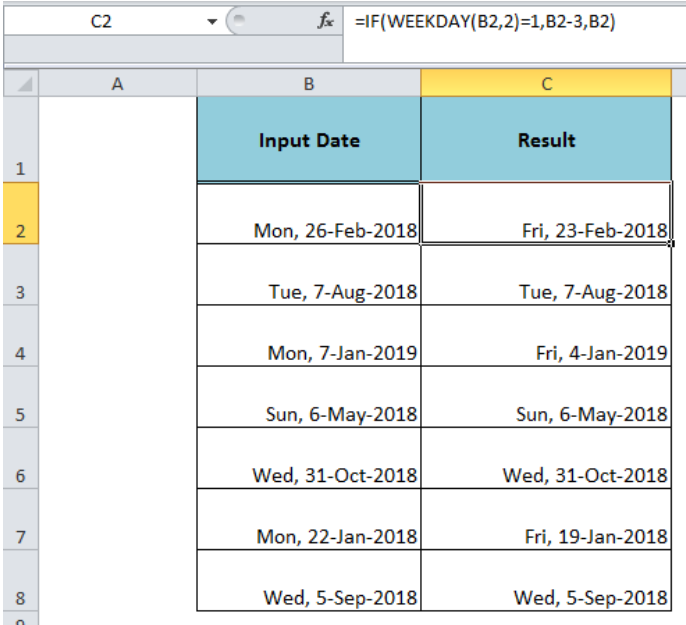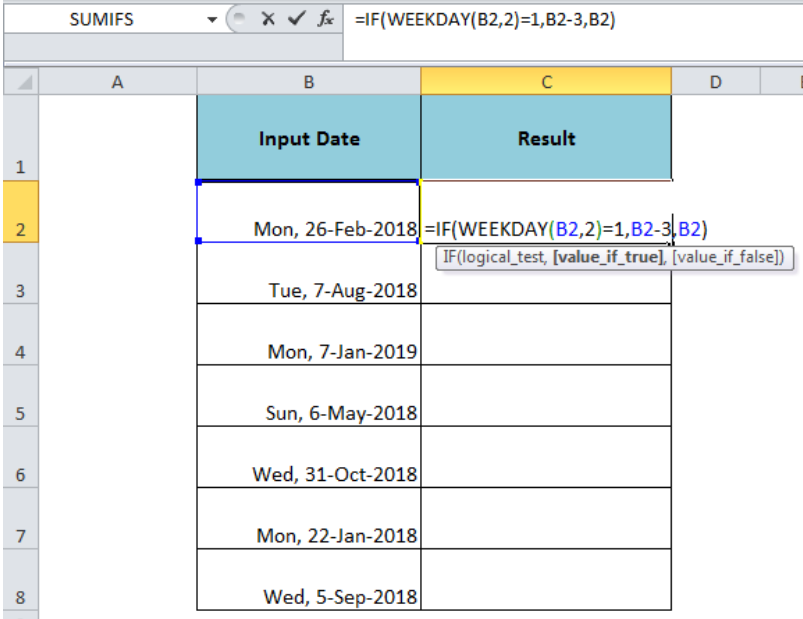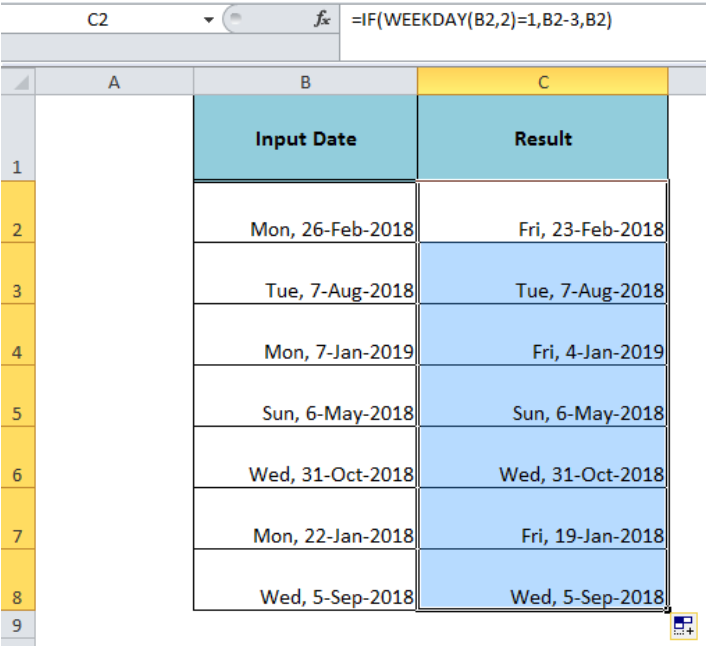Get instant live expert help with Excel or Google Sheets“My Excelchat expert helped me in less than 20 minutes, saving me what would have been 5 hours of work!”

#### Post your problem and you’ll get expert help in seconds.

Your message must be at least 40 characters
Our professional experts are available now. Your privacy is guaranteed.

# If Monday, roll back to FridayRead time: 20 minutes

When we enter the date and check the specified weekday for the date and want to roll it back to the required weekday, like check if Monday, roll back to Friday, then we need to use the Excel IF and WEEKDAY functions together in a formula.Figure 1. If Monday, Roll Back to Friday

## Formula Syntax

The generic syntax for the formula is;

`=IF(WEEKDAY(date,2)=1, date-3, date)`

Where,

• The Excel WEEKDAY function returns a number from 1 to 7 identifying the day of the week for a date according to the return-type index number, like for return_type index number 2, the WEEKDAY returns numbers 1 (Monday) through 7 (Sunday).
• Date – It is the date value entered as cell reference to check the weekday for.
• The IF function checks a logical condition and returns one value if the condition is TRUE, else the other value if the condition is FALSE.Figure 2. The Formula Syntax to Check If Monday, Roll Back to Friday

## Example

In the example, we have a list of date values in column B and will check if the weekday for the date is Monday, then roll it back to Friday date, else return the same date in column C. Using the above formula syntax, we will use the formula in cell C2 and copy it down, such as;

`=IF(WEEKDAY(B2,2)=1,B2-3,B2)`

The logical condition part of the formula, WEEKDAY(B2,2)=1, checks if the weekday for a date is equal to 1 (Monday), then subtract 3 days from the given date value (B2-3) to roll back to Friday date, else return the same date value.Figure 3. The Final Output of the Formula

## Instant Connection to an Expert through our Excelchat Service:

Most of the time, the problem you will need to solve will be more complex than a simple application of a formula or function. If you want to save hours of research and frustration, try our live Excelchat service! Our Excel Experts are available 24/7 to answer any Excel question you may have. We guarantee a connection within 30 seconds and a customized solution within 20 minutes.

Solution examplesCould you help me by explaining the IF function?
Solved by G. L. in 24 minsI have a question about Conditional Formatting. =IF('Sheet1'!A1="X",IF('Sheet1'!B1="Y", TRUE, FALSE),FALSE) the set the cell background to red. I would like to make it so that if Sheet1'!B1="Z" I set the back to blue. How can I achieve this.
Solved by B. F. in 39 minsI need to find out if the 2018 yearly sales goals were met if the yearly sales were \$25,000 or more using an IF logical function and to set the formula to return a value of YES if met, and NO if not
Solved by A. A. in 18 minsI filtered data in column A (it is labeled ID #) of a data set. On my next worksheet, column A is also the ID #, but it is a different data set. I want to filter the ID #'s the same for the two sheets, but how do I do that being that I am working with two different sets of data?
Solved by V. H. in 22 minsi want to create a very simple if function in a cell which is, if one cell is less than 1250, then the automatic value in the current cell is 250, dead easy, for someone who knows! Thank you.
Solved by A. H. in 14 mins